# RNA Sequence Analysis in R: edgeR

The purpose of this lab is to get a better understanding of how to use the edgeR package in R. http://www.bioconductor.org/packages/release/bioc/html/edgeR.html

We will largely be following their user manual.

If you haven't installed edgeR, you should run

source("http://bioconductor.org/biocLite.R")
biocLite("edgeR")


After you have installed edgeR, you load it like normal.

library(edgeR)


## Putting the data into the right format for edgeR

We'll work through an example dataset that is built into the package baySeq. This data set is a matrix (mobData) of counts acquired for three thousand small RNA loci from a set of Arabidopsis grafting experiments. baySeq is also a bioconductor package, and is also installed using

source("http://bioconductor.org/biocLite.R")
biocLite("baySeq")

library(baySeq)



##      SL236 SL260 SL237 SL238 SL239 SL240
## [1,]    21    52     4     4    86    68
## [2,]    18    21     1     5     1     1
## [3,]     1     2     2     3     0     0
## [4,]    68    87   270   184   396   368
## [5,]    68    87   270   183   396   368
## [6,]     1     0     6    10     6    12

#help(mobData)
mobDataGroups <- c("MM", "MM", "WM", "WM", "WW", "WW")
# MM="triple mutatnt shoot grafted onto triple mutant root"
# WM="wild-type shoot grafted onto triple mutant root"
# WW="wild-type shoot grafted onto wild-type root"
data(mobAnnotation)
#?mobAnnotation

##   chr start   end
## 1   1   789   869
## 2   1  8641  8700
## 3   1 10578 10599
## 4   1 17041 17098
## 5   1 17275 17318
## 6   1 17481 17527


edgeR works on a table of integer read counts, with rows corresponding to genes and columns to independent libraries. edgeR stores data in a simple list-based data object called a DGEList. This type of object is easy to use because it can be manipulated like any list in R. You can make this in R by specifying the counts and the groups in the function DGEList().

d <- DGEList(counts=mobData,group=factor(mobDataGroups))
d

## An object of class "DGEList"
## $counts ## SL236 SL260 SL237 SL238 SL239 SL240 ## 1 21 52 4 4 86 68 ## 2 18 21 1 5 1 1 ## 3 1 2 2 3 0 0 ## 4 68 87 270 184 396 368 ## 5 68 87 270 183 396 368 ## 2995 more rows ... ## ##$samples
##       group lib.size norm.factors
## SL236    MM   152461            1
## SL260    MM   309995            1
## SL237    WM   216924            1
## SL238    WM   208841            1
## SL239    WW   258404            1
## SL240    WW   276434            1


The philosophy of this package is that all the information should be contained in a single variable. When we use functions from this package later, we will write something like d <- estimateCommonDisp(d) which we normally would guess is overwriting d. Instead, this function passes everything that was already in d through the function but just adds one more element to the list.

## Filtering the data

First get rid of genes which did not occur frequently enough. We can choose this cutoff by saying we must have at least 100 counts per million (calculated with cpm() in R) on any particular gene that we want to keep. In this example, we're only keeping a gene if it has a cpm of 100 or greater for at least two samples.

dim(d)

##  3000    6

d.full <- d # keep the old one in case we mess up
head(d$counts)  ## SL236 SL260 SL237 SL238 SL239 SL240 ## 1 21 52 4 4 86 68 ## 2 18 21 1 5 1 1 ## 3 1 2 2 3 0 0 ## 4 68 87 270 184 396 368 ## 5 68 87 270 183 396 368 ## 6 1 0 6 10 6 12  head(cpm(d))  ## SL236 SL260 SL237 SL238 SL239 SL240 ## 1 137.740 167.745 18.44 19.15 332.81 245.990 ## 2 118.063 67.743 4.61 23.94 3.87 3.618 ## 3 6.559 6.452 9.22 14.36 0.00 0.000 ## 4 446.016 280.650 1244.68 881.05 1532.48 1331.240 ## 5 446.016 280.650 1244.68 876.26 1532.48 1331.240 ## 6 6.559 0.000 27.66 47.88 23.22 43.410  apply(d$counts, 2, sum) # total gene counts per sample

##  SL236  SL260  SL237  SL238  SL239  SL240
## 152461 309995 216924 208841 258404 276434

keep <- rowSums(cpm(d)>100) >= 2
d <- d[keep,]
dim(d)

##  724   6


This reduces the dataset from 3000 tags to about 700. For the filtered tags, there is very little power to detect differential expression, so little information is lost by filtering. After filtering, it is a good idea to reset the library sizes:

d$samples$lib.size <- colSums(d$counts) d$samples

##       group lib.size norm.factors
## SL236    MM   145153            1
## SL260    MM   294928            1
## SL237    WM   207227            1
## SL238    WM   200563            1
## SL239    WW   242628            1
## SL240    WW   259990            1


Note that the “size factor” from DESeq is not equal to the “norm factor” in the edgeR. In edgeR, the library size and additional normalization scaling factors are separated. See the two different columns in the $samples element of the 'DGEList' object above. In all the downstream code, the lib.size and norm.factors are multiplied together to act as the effective library size; this (product) would be similar to DESeq's size factor. ## Normalizing the data Read this short blog entry about normalizing RNA Seq data: http://www.rna-seqblog.com/data-analysis/which-method-should-you-use-for-normalization-of-rna-seq-data/ . edgeR normalizes by total count. edgeR is concerned with differential expression analysis rather than with the quantification of expression levels. It is concerned with relative changes in expression levels between conditions, but not directly with estimating absolute expression levels. The calcNormFactors() function normalizes for RNA composition by finding a set of scaling factors for the library sizes that minimize the log-fold changes between the samples for most genes. The default method for computing these scale factors uses a trimmed mean of M-values (TMM) between each pair of samples. We call the product of the original library size and the scaling factor the effective library size. The effective library size replaces the original library size in all downsteam analyses. d <- calcNormFactors(d) d  ## An object of class "DGEList" ##$counts
##   SL236 SL260 SL237 SL238 SL239 SL240
## 1    21    52     4     4    86    68
## 4    68    87   270   184   396   368
## 5    68    87   270   183   396   368
## 7    11    24    21    26    52    55
## 8   184   499   404   280   560   426
## 719 more rows ...
##
## $samples ## group lib.size norm.factors ## SL236 MM 145153 1.0285 ## SL260 MM 294928 0.8947 ## SL237 WM 207227 1.0128 ## SL238 WM 200563 0.7696 ## SL239 WW 242628 1.1946 ## SL240 WW 259990 1.1671  Without this, the default value is 1 for all values in d$samples$norm.factors. ## Data Exploration Before proceeding with the computations for differential expression, it is possible to produce a plot showing the sample relations based on multidimensional scaling. This is something that we will cover in much more detail in a later lecture. The basic premise is that we make a plot so samples which are similar are near to each other in the plot while samples that are dissimilar are far from each other. Here is an example. plotMDS(d, method="bcv", col=as.numeric(d$samples$group)) legend("bottomleft", as.character(unique(d$samples$group)), col=1:3, pch=20)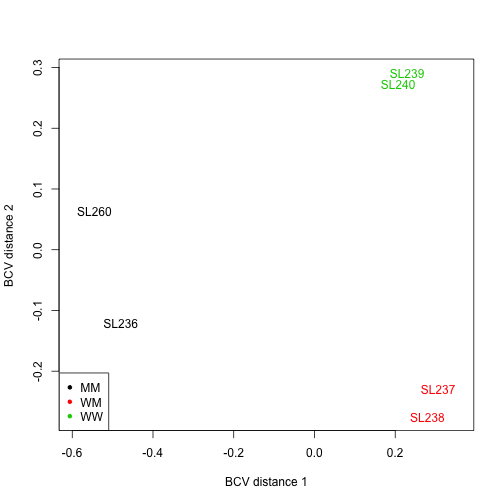Note that the different types separate out nicely. ## Estimating the Dispersion The first major step in the analysis of DGE data using the NB model is to estimate the dispersion parameter for each tag, a measure of the degree of inter-library variation for that tag. Estimating the common dispersion gives an idea of overall variability across the genome for this dataset. In this example, I am renaming the variable to d1 because we can estimate dispersion by assuming everything has the same common dispersion, or we can use a generalized linear model to try to estimate the dispersion. For now, we will just use the naive method of assuming all tags have the same dispersion. d1 <- estimateCommonDisp(d, verbose=T)  ## Disp = 0.07776 , BCV = 0.2789  names(d1)  ##  "counts" "samples" "common.dispersion" ##  "pseudo.counts" "pseudo.lib.size" "AveLogCPM"  For routine differential expresion analysis, we use empirical Bayes tagwise dispersions. Note that common dispersion needs to be estimated before estimating tagwise dispersions. For SAGE date, no abundance-dispersion trend is usually necessary. d1 <- estimateTagwiseDisp(d1) names(d1)  ##  "counts" "samples" "common.dispersion" ##  "pseudo.counts" "pseudo.lib.size" "AveLogCPM" ##  "prior.n" "tagwise.dispersion"  plotBCV() plots the tagwise biological coefficient of variation (square root of dispersions) against log2-CPM. plotBCV(d1)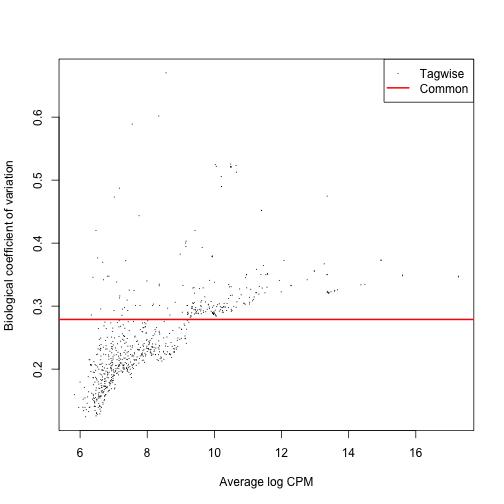Here we see that a single estimate for the coefficient of variation is a bad model since tagwise dispersion does not follow the model but instead increases as the counts per million (CPM) increases. ### GLM estimates of dispersion Fitting a model in edgeR takes several steps. First, you must fit the common dispersion. Then you need to fit a trended model (if you do not fit a trend, the default is to use the common dispersion as a trend). Then you can fit the tagwise dispersion which is a function of this model. In addition to the common and tagwise disperson, we can also estimate a generalized linear model (glm) fit using edgeR. In the same way that we've been doing above, we will just add these as additional data to the object we've been working with. design.mat <- model.matrix(~ 0 + d$samples$group) colnames(design.mat) <- levels(d$samples$group) d2 <- estimateGLMCommonDisp(d,design.mat) d2 <- estimateGLMTrendedDisp(d2,design.mat, method="power") # You can change method to "auto", "bin.spline", "power", "spline", "bin.loess". # The default is "auto" which chooses "bin.spline" when > 200 tags and "power" otherwise. d2 <- estimateGLMTagwiseDisp(d2,design.mat) plotBCV(d2)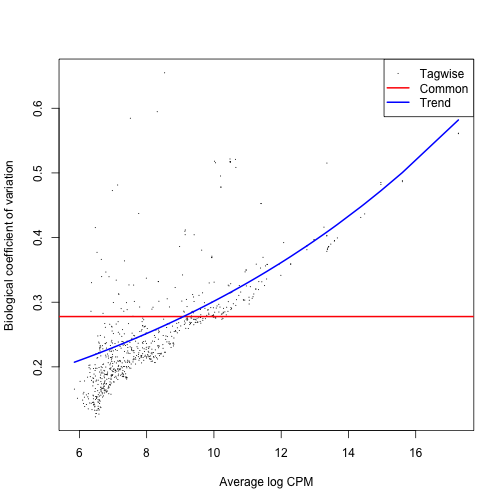Note that the tagwise biological coefficient of variation is different in this case than it was when we just estimated the common dispersion in the naive method above. This is because we model the tagwise dispersions based on the model derived from the glm model that we choose. If we change the method power above to something else, the tagwise errors change to reflect that the method is different. I chose the method="power" after looking at the methods that are offered: bin.spline (default if number of tags is > 200), power (default otherwise), bin.loess, and spline. We have 724 tags, so the default is bin.spline. When I used the bin.spline method, it was no better than estimating a common dispersion so I instead used power. ### Comparing the models in DESeq and edgeR DESeq always only uses a gamma glm as its model. Since edgeR does not have gamma glm as an option, we cannot produce the same glm results in edgeR as we can in DESeq and vice versa. Let's look at this same data using DESeq. require(DESeq) cds <- newCountDataSet( data.frame(d$counts), d$samples$group )
cds <- estimateSizeFactors( cds )
sizeFactors( cds )

##  SL236  SL260  SL237  SL238  SL239  SL240
## 0.6264 1.2336 0.9367 0.7361 1.4465 1.5078

cds <- estimateDispersions( cds , method="blind")
plotDispEsts(cds)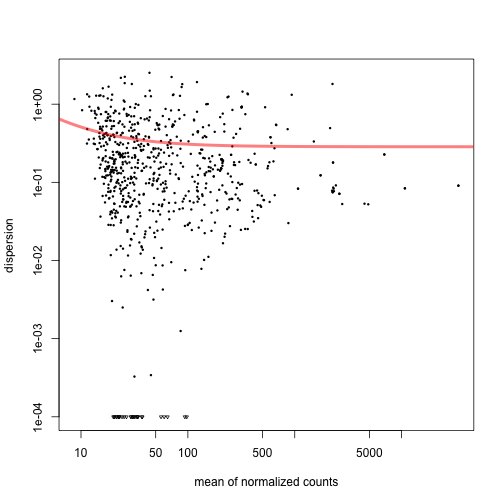Note that this plots dispersion on the vertical axis instead of the biological coefficient of variation.

### Dispersion and Biological Variation

The dispersion of a gene is simply another measure of a gene's variance and it is used by DESeq to model the overall variance of a gene's count values. The model for the variance $$v$$ of the count values used by DESeq is:

$v = s\mu + \alpha s^2 \mu^2 \ \text{ Where } \alpha \text{ is the dispersion, } \mu \text{ is the expected normalized count value and } s \text{ is the size factor}$

The dispersion can be interpreted as the square of the coefficient of biological variation (e.g. the difference in counts between two biological replicates is 40% so the gene's dispersion is $$0.4^2 = 0.16$$).

## Differential Expression

Once the dispersions are estimated, we can proceed with testing procedures for determining differential expression. The function exactTest() conducts tagwise tests using the exact negative binomial test. The test results for the n most significant tags are conveniently displayed by the topTags() function. By default, Benjamini and Hochberg's algorithm is used to control the false discovery rate (FDR).

Recall that d1 is the naive method where we only fit a common dispersion.

et12 <- exactTest(d1, pair=c(1,2)) # compare groups 1 and 2
et13 <- exactTest(d1, pair=c(1,3)) # compare groups 1 and 3
et23 <- exactTest(d1, pair=c(2,3)) # compare groups 2 and 3
topTags(et12, n=10)

## Comparison of groups:  WM-MM
##       logFC logCPM    PValue       FDR
## 74   10.430  9.124 2.729e-29 1.976e-26
## 1717  7.923  9.986 7.793e-29 2.821e-26
## 1111  9.670  8.360 1.062e-24 2.564e-22
## 1334  9.611  8.156 6.311e-24 1.142e-21
## 2322  5.174  8.667 3.070e-23 4.445e-21
## 625  -7.404  8.223 1.543e-22 1.862e-20
## 2537 -5.003  8.777 3.030e-22 3.133e-20
## 1353  9.033  7.697 8.618e-21 7.799e-19
## 1212 -4.760  9.119 2.819e-20 2.268e-18
## 2076  6.365  7.439 2.345e-18 1.698e-16

#topTags(et13, n=10)
#topTags(et23, n=10)


The total number of differentially expressed genes at FDR< 0:05 is:

de1 <- decideTestsDGE(et12, adjust.method="BH", p.value=0.05)
summary(de1)

##    [,1]
## -1  141
## 0   434
## 1   149


Here the entries for -1, 0 and 1 are for down-regulated, non-differentially expressed and up-regulated tags respectively.

The function plotSmear generates a plot of the tagwise log-fold-changes against log-cpm (analogous to an MA-plot for microarray data). DE tags are highlighted on the plot:

# differentially expressed tags from the naive method in d1
de1tags12 <- rownames(d1)[as.logical(de1)]
plotSmear(et12, de.tags=de1tags12)
abline(h = c(-2, 2), col = "blue")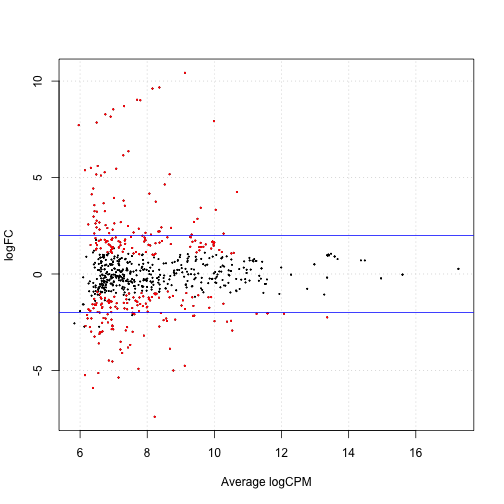### GLM testing for differential expression

Just as we used a GLM to fit the trend line above, we can also use this in finding the tags that are interesting by using a likelihood ratio test.

design.mat

##   MM WM WW
## 1  1  0  0
## 2  1  0  0
## 3  0  1  0
## 4  0  1  0
## 5  0  0  1
## 6  0  0  1
## attr(,"assign")
##  1 1 1
## attr(,"contrasts")
## attr(,"contrasts")$d$samples\$group
##  "contr.treatment"

fit <- glmFit(d2, design.mat)
# compare (group 1 - group 2) to 0:
# this is equivalent to comparing group 1 to group 2
lrt12 <- glmLRT(fit, contrast=c(1,-1,0))
lrt13 <- glmLRT(fit, contrast=c(1,0,-1))
lrt23 <- glmLRT(fit, contrast=c(0,1,-1))
topTags(lrt12, n=10)

## Coefficient:  1*MM -1*WM
##        logFC logCPM     LR    PValue       FDR
## 1717  -7.940  9.986 137.15 1.117e-31 8.085e-29
## 74   -10.663  9.126 124.46 6.671e-29 2.415e-26
## 1334  -9.844  8.155 116.85 3.103e-27 6.979e-25
## 1111  -9.903  8.362 116.41 3.856e-27 6.979e-25
## 625    7.446  8.230 104.86 1.313e-24 1.901e-22
## 1353  -9.266  7.700  97.56 5.235e-23 6.316e-21
## 2322  -5.183  8.666  94.71 2.206e-22 2.282e-20
## 2537   5.008  8.781  94.00 3.149e-22 2.850e-20
## 1696  -8.933  7.310  85.73 2.067e-20 1.662e-18
## 764   -9.242  7.797  84.31 4.235e-20 3.066e-18

#topTags(lrt13, n=10)
#topTags(lrt23, n=10)
de2 <- decideTestsDGE(lrt12, adjust.method="BH", p.value = 0.05)
de2tags12 <- rownames(d2)[as.logical(de2)]
plotSmear(lrt12, de.tags=de2tags12)
abline(h = c(-2, 2), col = "blue")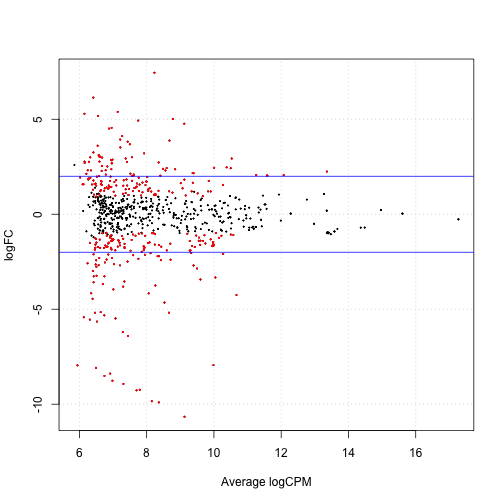# Questions

Answer the questions on OHMS “Homework 4: RNA seq”. Go to https://web.stanford.edu/class/bios221/cgi-bin/index.cgi/ to answer some questions. You may NOT work with other students to answer the questions on OHMS. You will need a Stanford ID to log in to OHMS.